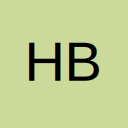# Vlookup or Index Match to populate multiple values

• Hi..
I am working on a file and trying to match the two columns. But thing is the entries in column 1 and column 2 are not unique. They are repeating, to avoid the confusion, I am taking help of another column containing Index values. Now I am using Index Match to populate the corresponding index value..But I am not getting the results..

## Files

• Re: Vlookup or Index Match to populate multiple values

Hi HB07,

Can you explain the logic behind this? How do you calculate what the desired results should look like?

• Re: Vlookup or Index Match to populate multiple values

Quote from S O;742035

Hi HB07,

Can you explain the logic behind this? How do you calculate what the desired results should look like?

Hi SO..
The logic is that there are certain values in Column A which are repeating and there are certain values in B which are also repeating.. Now I want to match How many values in A and B are matching..So for this I took the help of an helping column which has got the index values starting from 1 to n.The index values are placed manually.. Now the idea is to populate the index value when the value in A matches with the value in B..For example,if you look at the snapshot..the value in A16 which is "D" matched with value in B13..So I have populated the index value of B13 in E16...and so on.

Please feel free to let me know..if my question sounds confusing.

Also let me know, if you are able to get the logic for the same

• Re: Vlookup or Index Match to populate multiple values

=IF(COUNTIF(\$B\$2:B2,A2)<COUNTIF(\$A\$2:A2,A2),"",INDEX(\$C\$2:\$C\$19,MATCH(A2,\$B\$2:\$B\$19,0)+COUNTIF(\$A\$2:A2,A2)-1))

that formula works on your test data

## Participate now!

Don’t have an account yet? Register yourself now and be a part of our community!